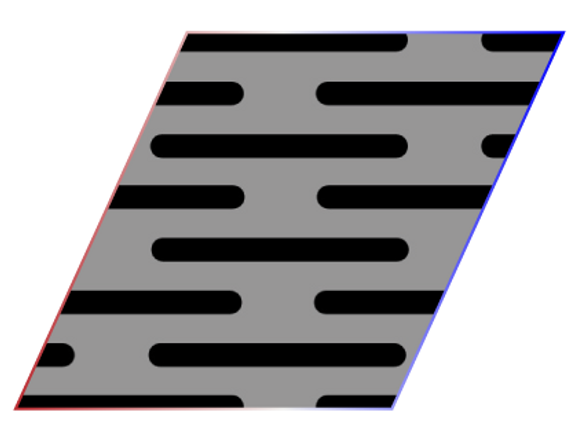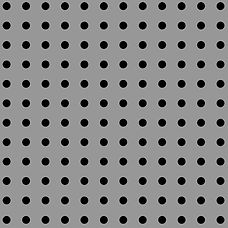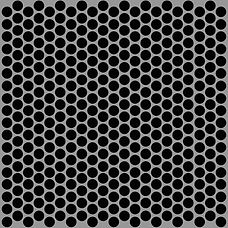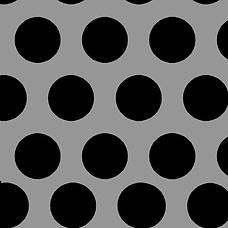# Standard Holes## DM_SR_101

Square (Pitch : Rectangular)

• Hole size ( C ) = 8mm

• Pitch 1 ( Z1) = 8mm

• Pitch 2 ( Z2) = 8mm

• Open Area = 22%## DM_OS_105

Oblong Staggered

• Hole Length (L) = 30mm

• Hole Width ( R ) = 16mm

• Pitch 1 ( Z 1) = 48mm

• Pitch 2 ( Z2 ) = 48mm

• Open Area = 36.90%## DM_OS_102

Oblong Staggered

• Hole Length (L) = 20mm

• Hole Width ( R ) = 2mm

• Pitch 1 ( Z 1) = 8mm

• Pitch 2 ( Z2 ) = 20mm

• Open Area = 39.10%## DM_OS_103

Oblong Square

• Hole Length (L) = 38 mm

• Hole Width ( R ) = 6 mm## DM_HS_104

Hexagon Staggered

• Hole Size ( H ) = 24 mm

• Pitch ( T ) = 30 mm

• Open Area = 64%## DM_HS_101

Hexagon Staggered

• Hole Length ( L ) = 40 mm

• Hole Width ( C ) = 12 mm

• Pitch 1 ( Z1) = 40 mm

• Pitch 2 ( Z2) = 48 mm## DM_RS_101

Rectangle Staggered

• Hole Length ( L ) = 16 mm

• Hole Width ( C ) = 5.4 mm

• Pitch 1 ( Z1) = 16 mm

• Pitch 2 ( Z2) = 20 mm## DM_RT_1014

Round Staggered

• Hole Diameter (R) = 6.35 mm

• Pitch (U1) = 9.35 mm

• Open Area = 40%## DM_RT_1011

Round Staggered

• Hole Diameter (R) = 3.17 mm

• Pitch (U1) = 4.67 mm

• Open Area = 41.80%## DM_RT_101

Round Staggered

• Hole Diameter (R) = 10 mm

• Pitch (U1) = 15 mm

• Open Area =40%## DM_RT_105

Round Staggered

• Hole Diameter (R) = 28 mm

• Pitch (U1) = 35 mm

• Open Area = 58%## DM_RT_102

Round Staggered

• Hole Diameter (R) = 2.8 mm

• Pitch (U1) = 5.8 mm

• Open Area = 21.10%## DM_RR_104

Round Rectangle

• Hole Diameter (R) = 6.35mm

• Pitch (U1) = 5mm

• Open Area = 126.60%## DM_RR_102

Round Rectangle

• Hole Diameter (R) = 3mm

• Pitch (U1) = 8mm

• Open Area = 11%## DM_SS_101

Square (Pitch : Staggered)

• Hole size ( C ) = 8mm

• Pitch 1 ( Z1) = 8mm

• Pitch 2 ( Z2) = 8mm

• pen Area = 44%## DM_OS_104

Oblong Staggered

• Hole Length (L) = 40mm

• Hole Width ( R ) = 8mm

• Pitch 1 ( Z 1) = 13mm

• Pitch 2 ( Z2 ) = 82mm

• Open Area = 57.50%## DM_OS_101

Oblong Staggered

• Hole Length (L) = 20 mm

• Hole Width ( R ) = 1.5 mm

• Pitch 1 ( Z 1) = 7.5 mm

• Pitch 2 ( Z2 ) = 25 mm

• Open Area = 31.50%## DM_OS_102

Oblong Square

• Hole Length (L) = 25 mm

• Hole Width ( R ) = 3 mm## DM_HS_103

Hexagon Staggered

• Hole Size ( H ) = 9 mm

• Pitch ( T ) = 12 mm

• Open Area = 56.25%## DM_RS_103

Rectangle Staggered

• Hole Length ( L ) = 40 mm

• Hole Width ( C ) = 12 mm

• Pitch 1 ( Z1) = 40 mm

• Pitch 2 ( Z2) = 48 mm## DM_RT_1016

Round Staggered

• Hole Diameter (R) = 1.5 mm

• Pitch (U1) = 4.3 mm

• Open Area = 11%## DM_RT_1013

Round Staggered

• Hole Diameter (R) = 8 mm

• Pitch (U1) = 10 mm

• Open Area =58%## DM_RT_1010

Round Staggered

• Hole Diameter (R) = 12 mm

• Pitch (U1) = 16 mm

• Open Area = 51%## DM_RT_107

Round Staggered

• Hole Diameter (R) = 6 mm

• Pitch (U1) = 7.5 mm

• Open Area = 0.58%## DM_RT_104

Round Staggered

• Hole Diameter (R) = 10 mm

• Pitch (U1) = 15 mm

• Open Area = 40.30%## DM_RR_106

Round Rectangle

• Hole Diameter (R) = 4.5mm

• Pitch (U1) = 12.6mm

• Open Area = 10%## DM_RR_103

Round Rectangle

• Hole Diameter (R) = 4mm

• Pitch (U1) = 8mm

• Open Area = 19.60%## DM_OS_106

Oblong Staggered

• Hole Length (L) = 25mm

• Hole Width ( R ) = 18mm

• Pitch 1 ( Z 1) = 35mm

• Pitch 2 ( Z2 ) = 43mm

• Open Area = 50.50%## DM_OS_103

Oblong Staggered

• Hole Length (L) = 25mm

• Hole Width ( R ) = 2mm

• Pitch 1 ( Z 1) = 16.5mm

• Pitch 2 ( Z2 ) = 25mm

• Open Area = 23.80%## DM_OS_104

Oblong Square

• Hole Length (L) = 35 mm

• Hole Width ( R ) = 12 mm## DM_OS_101

Oblong Square

• Hole Length (L) = 20 mm

• Hole Width ( R ) = 2.5 mm## DM_HS_102

Hexagon Staggered

• Hole Size ( H ) = 6 mm

• Pitch ( T ) = 9 mm

• Open Area = 44.40%## DM_RS_102

Rectangle Staggered

• Hole Length ( L ) = 10 mm

• Hole Width ( C ) = 5 mm

• Pitch 1 ( Z1) = 14 mm

• Pitch 2 ( Z2) = 20 mm## DM_RT_1015

Round Staggered

• Hole Diameter (R) = 1.5 mm

• Pitch (U1) = 3.36 mm

• Open Area = 18%## DM_RT_1012

Round Staggered

• Hole Diameter (R) = 5 mm

• Pitch (U1) = 8 mm

• Open Area =35%## DM_RT_109

Round Staggered

• Hole Diameter (R) = 8 mm

• Pitch (U1) = 12 mm

• Open Area = 40%## DM_RT_106

Round Staggered

• Hole Diameter (R) = 20 mm

• Pitch (U1) = 25 mm

• Open Area = 58%## DM_RT_103

Round Staggered

• Hole Diameter (R) = 3.1 mm

• Pitch (U1) = 4.8 mm

• Open Area = 37.80%## DM_RR_105

Round Rectangle

• Hole Diameter (R) = 22mm

• Pitch (U1) = 25mm

• Open Area = 60.80%## DM_RR_101

Round Rectangle

• Hole Diameter (R) = 1.2 mm

• Pitch (U1) = 4mm

• Open Area = 7.10%